Question

A population of 1,000 students spends an average of \$10.50 a day on dinner. The standard deviation of the expenditure is \$3. A simple random sample of 64 students is taken.

a.         What are the expected value, standard deviation, and shape of the sampling distribution of the sample mean?

Answer: 10.5     0.363   normal

b. What is the probability that these 64 students will spend a combined total between \$10.99 and \$11.38?

c. What is the probability that these 64 students will spend a combined total of more than 11.18?

Solution :

Given that,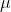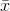= 10.5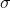= 0.363

b)  P(10.99 << 11.38)

= P[(10.99 - 10.5) /0.363 < (-) /< (11.38 - 10.5) / 0.363)]

= P(1.35 < Z < 2.42)

= P(Z < 2.42) - P(Z < 1.35)

Using z table,

= 0.9922 - 0.9115

= 0.0807

c) P(> 11.18) = 1 - P(< 11.18)

= 1 - P[(-) /< (11.18 - 10.5) /0.363 ]

= 1 - P(z < 2.42)

Using z table,

= 1 - 0.9922

= 0.0078

#### Earn Coins

Coins can be redeemed for fabulous gifts.

Similar Homework Help Questions
• ### Sampling Distribution 1. A population of 1,000 students spends an average of \$10.50 a day on...

Sampling Distribution 1. A population of 1,000 students spends an average of \$10.50 a day on dinner. The standard deviation of the expenditure is \$3. A simple random sample of 64 students is taken. A. What is the expected value, standard deviation, and shape of the sampling distribution of the sample mean? B. What is the probability that these 64 students will spend a combined total of more than \$715.21? C. What is the probability that these 64 students will...

• ### 2. (14 pts) A population of 1,000 students spends an average of \$10.50 a day on...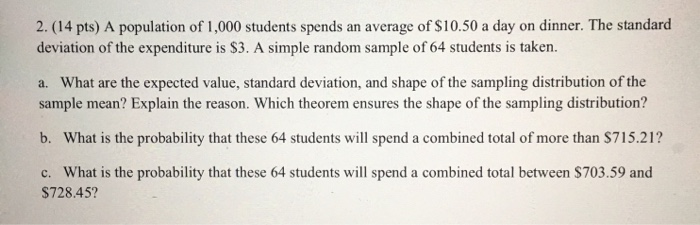2. (14 pts) A population of 1,000 students spends an average of \$10.50 a day on dinner. The standard deviation of the expenditure is \$3. A simple random sample of 64 students is taken. a. What are the expected value, standard deviation, and shape of the sampling distribution of the sample mean? Explain the reason. Which theorem ensures the shape of the sampling distribution? b. What is the probability that these 64 students will spend a combined total of more...

• ### sampling

1. A population of 1,000 students spends an average of \$10.50 a day on dinner. The standard deviation of the expenditure is \$3. A simple random sample of 64 studentsis taken.a. What is the expected value, standard deviation, and shape of the sampling distribution of the sample mean?b. What is the probability that these 64 students will spend a combined total of more than \$715.21?c. What is the probability that these 64 students will spend a combined total between \$703.59...

• ### Do i use the formula for finite or infinite? It gives me a population size so...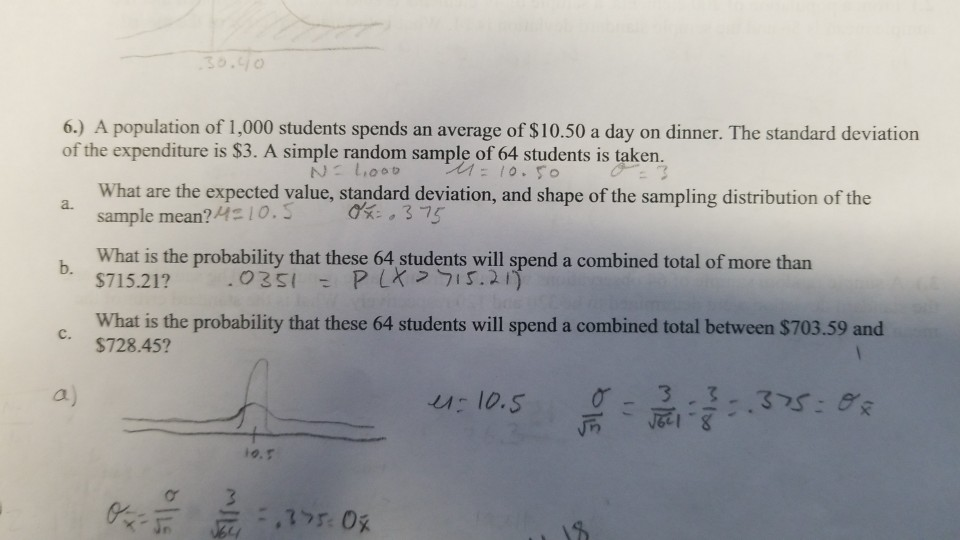Do i use the formula for finite or infinite? It gives me a population size so i was thinking finite, but everyone seems to going with the infinite formula to find the Standard deviation? 6.) A population of 1,000 students spends an average of \$10.50 a day on dinner. The standard deviation of the expenditure is S3. A simple random sample of 64 students is taken. What are the expected value, standard deviation, and shape of the sampling distribution of...

• ### Find the probability

A population of 1,000 students spends an average of \$10.50 a day on dinner. The standard deviation of the expenditure is \$3. A simple random sample of 64 students istaken.b. What is the probability that these 64 students will spend a combined total of more than \$715.21?c. What is the probability that these 64 students will spend a combined total between \$703.59 and \$728.45?

• ### question

A population of 1,000 students spends an average of \$10.50 a day on dinner. The standard deviation of the expenditure is \$3. A simple random sample of 64 students istaken

• ### Students of a large university spend an average of \$5 a day on lunch. The standard...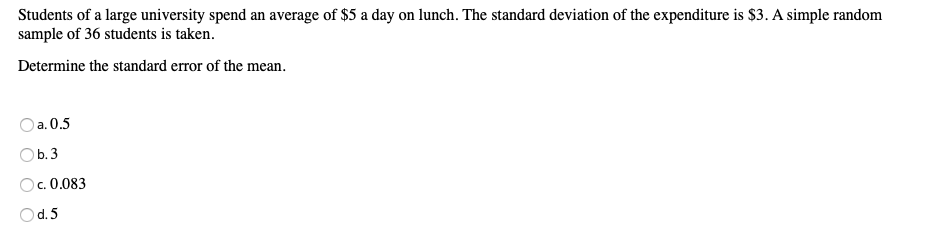Students of a large university spend an average of \$5 a day on lunch. The standard deviation of the expenditure is \$3. A simple random sample of 36 students is taken. Determine the standard error of the mean. a. 0.5 Ob.3 c. 0.083 d. 5 Students of a large university spend an average of \$5 a day on lunch. The standard deviation of the expenditure is \$3. A simple random sample of 36 students is taken. What is distribution of...

• ### Econ 10 worked out answers

Students of a large university spend an average of \$5 a day on lunch. The standarddeviation of the expenditure is \$3. A simple random sample of 36 students is taken.a. What are the expected value and standard deviation of the sampling distributionof the sample mean?b. What is the probability that the sample mean will be at least \$4?c. What is the probability that the sample mean will be at least \$5.90?

• ### 10. The population average for SAT score is 1020, with a population standard deviation of 100...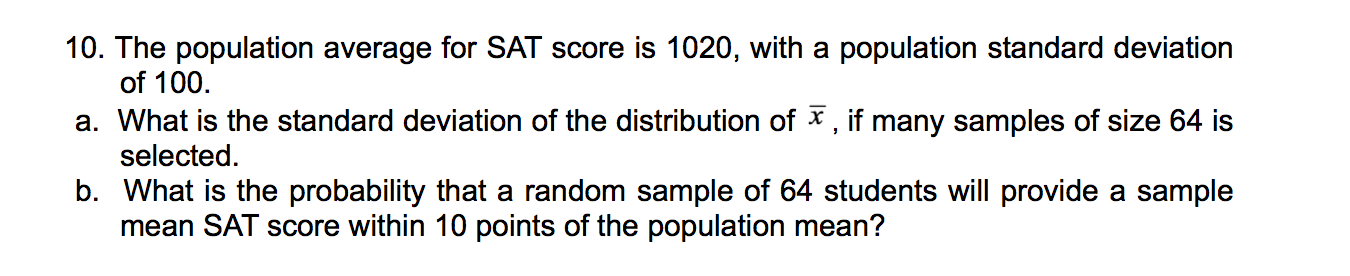10. The population average for SAT score is 1020, with a population standard deviation of 100 a. What is the standard deviation of the distribution of , if many samples of size 64 is selected b. What is the probability that a random sample of 64 students will provide a sample mean SAT score within 10 points of the population mean?

• ### 9. Suppose that the average festival attendee spends \$1100 for the entire Coachella experience with standard...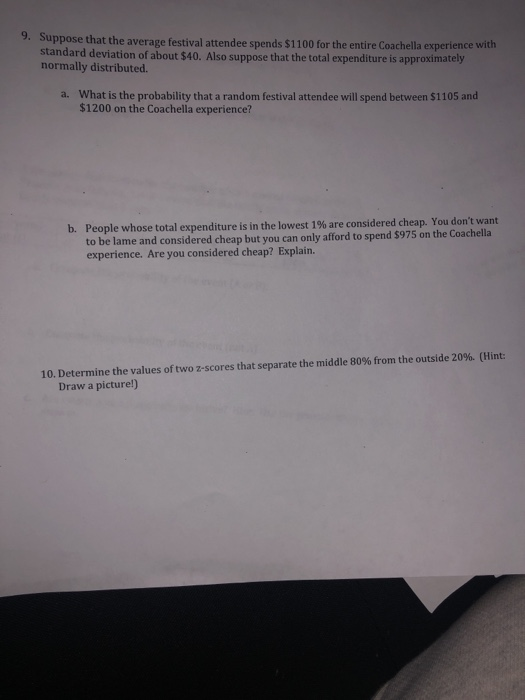9. Suppose that the average festival attendee spends \$1100 for the entire Coachella experience with standard deviation of about \$40. Also suppose that the total expenditure is approximately normally distributed. What is the probability that a random festival attendee will spend between \$1105 and \$1200 on the Coachella experience? a. on the Coadheila sperien People whose total expenditure is in the lowest 196 are considered cheap. You don't want to be lame and considered cheap but you can only afford...